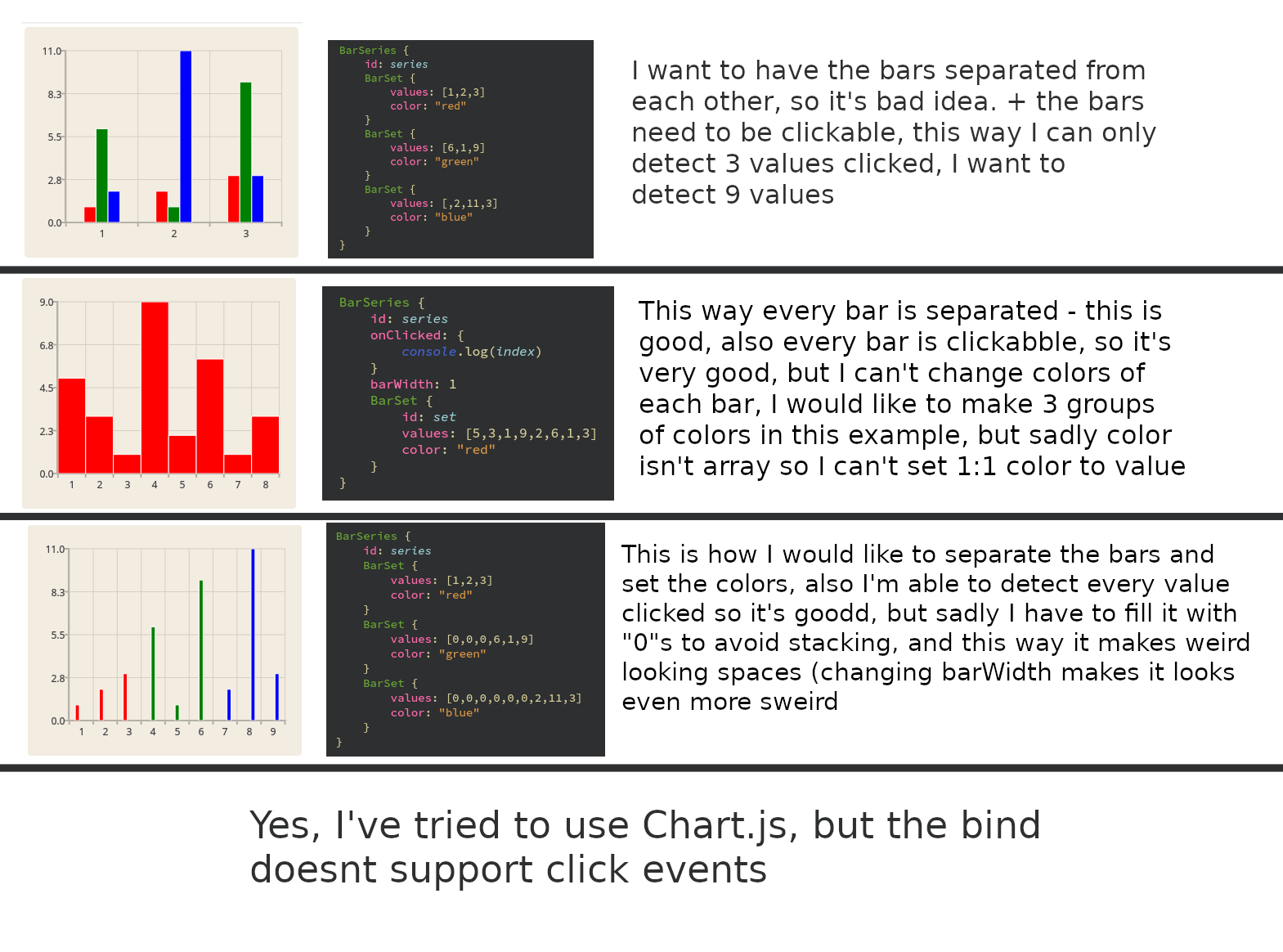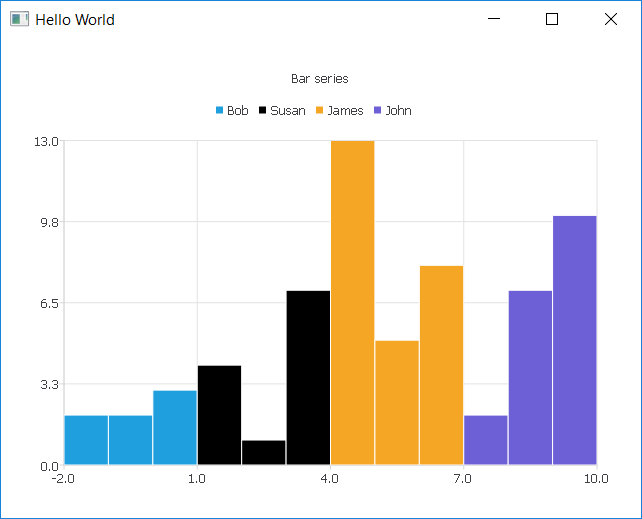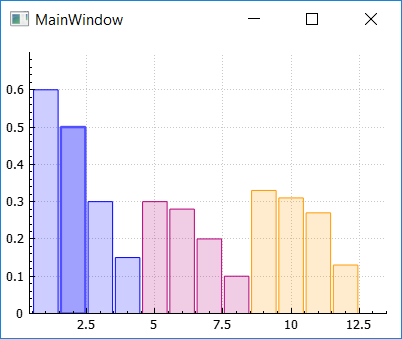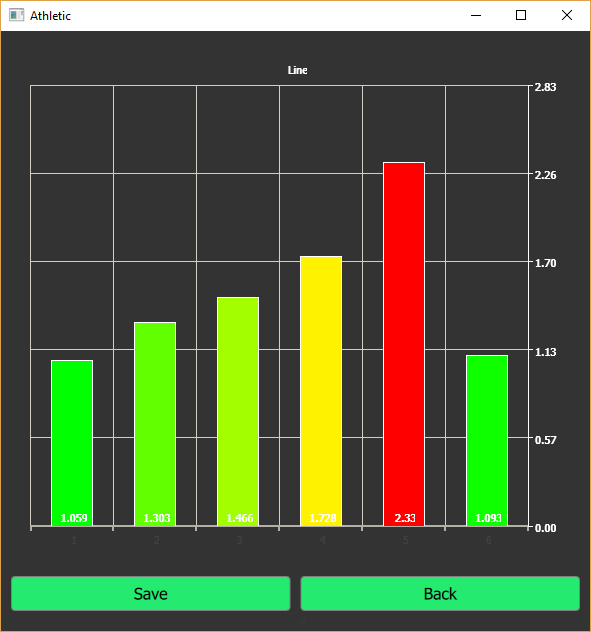# Is there way to create the simplest bar chart?

• Hey, I'm trying to reach the simplest bar chart, but I'm having so many problems, look at the picture, so you'll be able to understand my problem, it's a little bit cunfused problem• Hi @orzel1244
What are you trying to do when you click?

In the following example (from the Qt QML Charts example), you have 3 series laid out as you wanted:
Then, to test the click I added the `onClicked` handler, in which I use the attached `index` property of each `BarSet` to get the clicked value:

``````    ChartView {
title: "Bar series"
anchors.fill: parent
legend.alignment: Qt.AlignBottom
antialiasing: true

BarSeries {
id: mySeries
axisX: BarCategoryAxis { categories: ["2007", "2008", "2009", "2010", "2011", "2012" ] }
BarSet {
label: "Bob"
values: [2, 2, 3, 4, 5, 6]
onClicked: console.debug("Bob clicked!" + index + " " + this.at(index))
}
BarSet {
label: "Susan"
values: [5, 1, 2, 4, 1, 7]
onClicked: console.debug("Susan clicked!" + index + " " + this.at(index))
}
BarSet {
label: "James"
values: [3, 5, 8, 13, 5, 8]
onClicked: console.debug("James clicked!" + index + " " + this.at(index))
}
}
}
``````

So each item in each of the bar sets is individually clickable and its value accessible, which is what I suppose you are trying to achieve, since I assume you are trying to show the value when hovered.

• The thing is, I dont want to stack these bars, I want to achive result from picture 2 and 3, I mean every bar is inddependent, and the bars have individual colors, It's nice to know that I can make every bar clickable on this first image, but it doesn't help me much, because I don't want to stack the bars like that.

Basicly I want to achive result from second picture, but with ability to change color of each bar

• @orzel1244
My bad... didn't read everything through thoroughly enough... What you are trying to achieve seems indeed not easily done in QML and it is a pity that the 3rd approach does not provide an easy way to play with the `barWidth` property.

For what it is worth, I played around and found you can close the gap between the series as follows:

1. Use the `Qt.point(index, datavalue)` approach for setting the `values` of each `BarSet`,
2. Let the first index of the next series coincide with the last index of the previous series
3. Set `BarSeries::barWidth` to `BarSeries::count`

This however completely closes the gap between the bars, but the border of each bar gives a small separation effect.

Furthermore, you need to play with the x-axis limits, to get everything into view, which may lead to negative minimum x, which depends on the number of series you add etc...

For example:

``````    ChartView {
title: "Bar series"
anchors.fill: parent
antialiasing: true

BarSeries {
id: mySeries
barWidth: mySeries.count

axisX: ValueAxis  {
min: -2
max: 10
}

BarSet {
label: "Bob"
values: [Qt.point(0, 2), Qt.point(1, 2), Qt.point(2, 3)];
onClicked: console.debug("Bob clicked!" + index + " " + this.at(index))
}

BarSet {
label: "Susan"
values: [Qt.point(2, 4), Qt.point(3, 1), Qt.point(4, 7)];
color: "black"
onClicked: console.debug("Susan clicked!" + index + " " + this.at(index))
}

BarSet {
label: "James"
values: [Qt.point(4, 13), Qt.point(5, 5), Qt.point(6, 8)];
onClicked: console.debug("James clicked!" + index + " " + this.at(index))
}

BarSet {
label: "John"
values: [Qt.point(6, 2), Qt.point(7, 7), Qt.point(8, 10)];
onClicked: console.debug("John clicked!" + index + " " + this.at(index))
}
}
}
``````Not very practical all that, of course...

Otherwise, if you don't mind the C++ way, you could try `QCustomPlot` which is very easy to include in your project, but somewhat difficult to get to learn.
https://www.qcustomplot.com/

//MainWindow.h

``````#include "qcustomplot.h"

namespace Ui {
class MainWindow;
}

class MainWindow : public QMainWindow
{
Q_OBJECT

public slots:
void onPlottableClick(QCPAbstractPlottable*,int,QMouseEvent*);

public:
explicit MainWindow(QWidget *parent = 0);
~MainWindow();

private:
Ui::MainWindow *ui;
QCustomPlot* m_customplot;
};
``````

//MainWindow.cpp

``````MainWindow::MainWindow(QWidget *parent) :
QMainWindow(parent),
ui(new Ui::MainWindow)
{
ui->setupUi(this);

m_customplot = new QCustomPlot(this);
m_customplot->setInteraction(QCP::iSelectPlottables, true);
m_customplot->setInteraction(QCP::iSelectItems, true);

m_customplot->setMinimumHeight(300);
m_customplot->setMinimumWidth(400);

QVector<double> datax1 = QVector<double>() << 1 << 2 << 3 << 4;
QVector<double> datay1 = QVector<double>() << 0.6 << 0.5 << 0.3 << 0.15;

QVector<double> datax2 = QVector<double>() << 1 << 2 << 3 << 4 << 5 << 6 << 7 << 8;
QVector<double> datay2 = QVector<double>() << 0.0 << 0.0 << 0.0 << 0.0 << 0.3 << 0.28 << 0.2 << 0.1;

QVector<double> datax3 = QVector<double>() << 1 << 2 << 3 << 4 << 5 << 6 << 7 << 8 << 9 << 10 << 11 << 12;
QVector<double> datay3 = QVector<double>() << 0.0 << 0.0 << 0.0 << 0.0 << 0.0 << 0.0 << 0.0 << 0.0 <<0.33 << 0.31 << 0.27 << 0.13;

double bwidth = 0.9;

connect(m_customplot, SIGNAL(plottableClick(QCPAbstractPlottable*,int,QMouseEvent*)), this, SLOT(onPlottableClick(QCPAbstractPlottable*,int,QMouseEvent*)));

QCPBars *bars = new QCPBars(m_customplot->xAxis, m_customplot->yAxis);
bars->setSelectable(QCP::stSingleData);
bars->setData(datax1, datay1);
bars->setBrush(QColor(0, 0, 255, 50));
bars->setPen(QColor(0, 0, 255));
bars->setWidth(bwidth);
bars->setWidthType(QCPBars::wtPlotCoords);

bars = new QCPBars(m_customplot->xAxis, m_customplot->yAxis);
bars->setSelectable(QCP::stSingleData);
bars->setData(datax2, datay2);
bars->setBrush(QColor(180, 00, 120, 50));
bars->setPen(QColor(180, 00, 120));
bars->setWidthType(QCPBars::wtPlotCoords);
bars->setWidth(bwidth);

bars = new QCPBars(m_customplot->xAxis, m_customplot->yAxis);
bars->setSelectable(QCP::stSingleData);
bars->setData(datax3, datay3);
bars->setBrush(QColor(255, 154, 0, 50));
bars->setPen(QColor(255, 154, 0));
bars->setWidthType(QCPBars::wtPlotCoords);
bars->setWidth(bwidth);

m_customplot->xAxis->setRange(0.4, 13.5);
m_customplot->yAxis->setRange(0, 0.7);
}

MainWindow::~MainWindow()
{
delete ui;
}

void MainWindow::onPlottableClick(QCPAbstractPlottable * plottable, int dataIndex, QMouseEvent * event)
{
qDebug() << "Clicked: " << dataIndex;
}
``````Although not easy to use, `QCustomPlot` is highly customizable, and since the full source code is contained in only 2 files, you can even modify it on the fly according to your needs.

If that works for you, then the next step would be to import the plot in QML, see e.g;
https://www.qcustomplot.com/index.php/support/forum/172

Good luck.

• It's not all I need, but it's really close to effect which I want to achive, thank you so much :)
Solved.

• Even though this topic is old I want to share the solution which works for me with qt 5.14.

I had used the Qt Quick approach from orzel1244 , and by the way want to say thanks him. But afterwords, I managed to solve the "separete color for each bar" topic with `StackedBarSeries` and some modifications. Final result is bellow :

`````` StackedBarSeries {
id: barSeries
axisX: BarCategoryAxis {
id: categoriesAxis
}

axisYRight: valueAxisY //customize it yourself
labelsVisible: true
labelsPosition: AbstractBarSeries.LabelsInsideBase
}
///////////////////////////
function setPoints(pointsTime) {
barSeries.clear()
categoriesAxis.clear()
for (var i = 0; i < pointsTime.length; i++)
{
barSeries.append(i, [Qt.point(i, pointsTime[i])])
barSeries.at(i).color = calculateColor(...)
categoriesAxis.categories.push(i+1)
}
}
``````